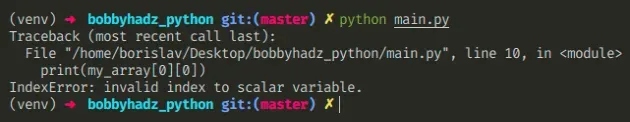# IndexError: invalid index to scalar variable in PythonFri Apr 22 20222 min readPhoto by Ikk Bahctiar

## IndexError: invalid index to scalar variable in Python#

The Python "IndexError: invalid index to scalar variable" occurs when we try to access a numpy scalar like an integer or a float at a specific index. To solve the error, make sure the value you are trying to index is an array or another sequence with the right dimensions.Here is an example of how the error occurs.

main.py
```Copied!```import numpy as np

my_array = np.array([1, 2, 3])

print(my_array)  # 👉️ 1

# ⛔️ IndexError: invalid index to scalar variable.
print(my_array)
``````

We accessed the array element at index `0`, which has a value of `1`.

Then, we tried to access `1` at index `0` which caused the error.

We can't access a numpy scalar like an integer or a float at a specific index.

If you meant to access an item in an array, make sure the variable is an array and use a single set of square brackets.

main.py
```Copied!```import numpy as np

my_array = np.array([1, 2, 3])

print(my_array)  # 👉️ 1
print(my_array)  # 👉️ 2
print(my_array)  # 👉️ 3
``````

If you meant to declare a two-dimensional array, use the following syntax.

main.py
```Copied!```import numpy as np

my_array = np.array([[1, 2, 3], [4, 5, 6]])

print(my_array)  # 👉️ 1
print(my_array)  # 👉️ 2
print(my_array)  # 👉️ 3
``````

We declared a two-dimensional array (an array of arrays).

The first set of square brackets gives us access to the first nested array (index `0`).

The second set of square brackets allows us to access items in the nested array.

Print the variable you are trying to access at a specific index and make sure it contains what you expect.

main.py
```Copied!```import numpy as np

my_array = np.array([[1, 2, 3], [4, 5, 6]])

print(my_array) # 👉️ [[1 2 3] [4 5 6]]
``````

A common cause of the error is reassigning a variable that stores an array to a scalar (an int or a float) before accessing the value at an index.

main.py
```Copied!```import numpy as np

my_array = np.array([[1, 2, 3], [4, 5, 6]])

# 👇️ reassign variable by mistake
my_array = np.int32(100)

# ⛔️ IndexError: invalid index to scalar variable.
print(my_array)
``````

We reassigned the array to an integer before trying to access the value at a specific index which caused the error.

Use the search field on my Home Page to filter through my more than 1,000 articles.